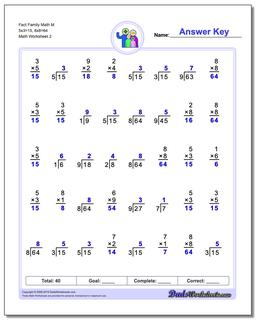PLEASE GO BACK AND USE THE BIG BLUE 'PRINT' BUTTON ON THE PAGE TO PRINT THE WORKSHEET CORRECTLY!Sorry for the trouble! The browser won't print the embedded worksheet PDF directly using the normal 'Print' command in the file menu, so you need to click the big 'Print' button to send just the worksheet and not the surrounding page to the printer.

# Math Worksheets: Fact Family Math: Fact Family Math: Fact Family Math M 5x3=15, 8x8=64 (Second Worksheet)## Fact Family Math M 5x3=15, 8x8=64 (Second Worksheet)

PropertyValue
DescriptionFact Family Math M 5x3=15, 8x8=64: These 40 problem multiplication and division fact family worksheets are perfect for either quick practice or a one minute timed test. For help on individual operations, check out the multiplication worksheets and division worksheets pages! (Second Worksheet)
Resource TypeWorksheet